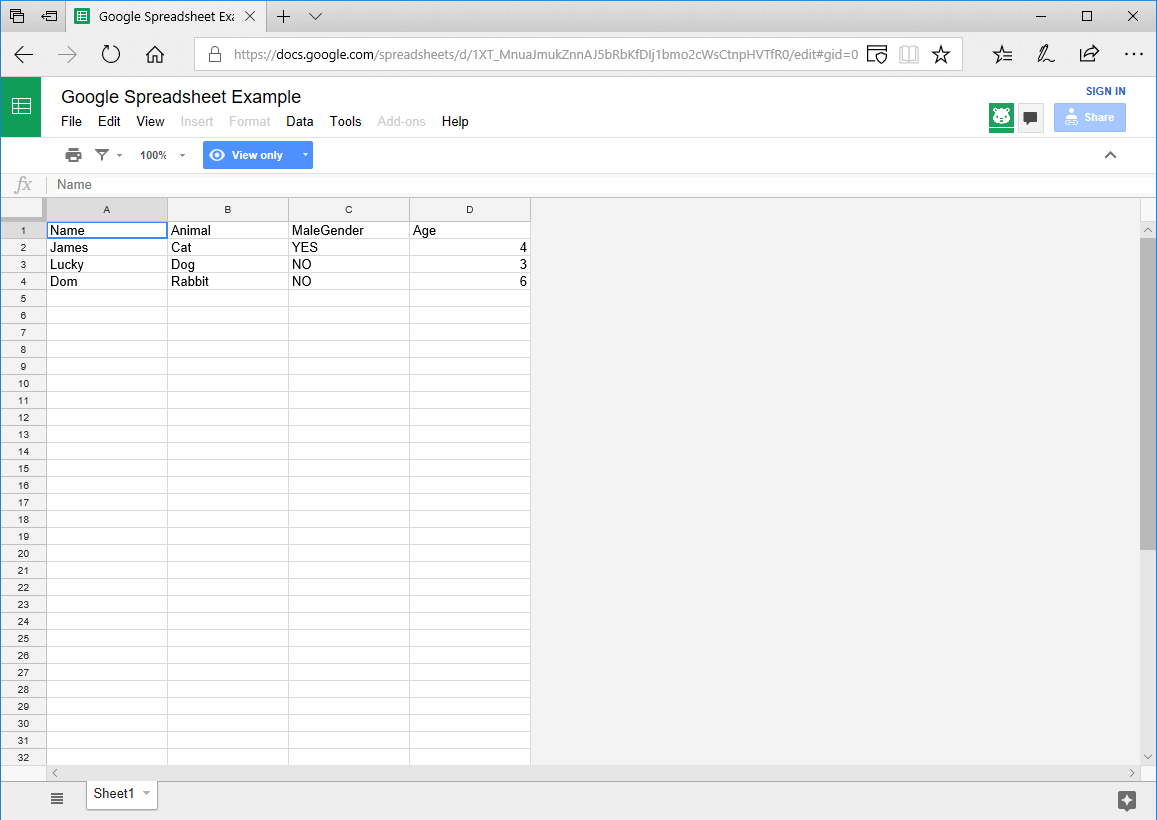To simplify collaborators working with iOS development, I’ve decided that Google Spreadsheet is a good way of handling simple data collection. Which would then be exported to XML format for download, useful for updating applications on the fly.

• Edit spreadsheet in real time, no modification conflict
• No learning of XML or PLIST
• Set validation for each cell, restrict user error
• User interface
• Require PHP server

Google has removed support for outputting RSS which was useful in creating XML. But there is an tutorial in exporting using CSV:

Rob has provided an brilliant example converting CSV into array, which can be used to convert it into JSON, XML, PLIST format

Would this be useful for your project?, can just check out this online example.

Encode:

csv.php
Use to retrieve the Google CSV file, separating each cell into arrays.

```&amp;lt;?php
class CSV {
// Function to convert CSV into associative array
public function getArray(\$file, \$delimiter = ",") {
if ((\$handle = fopen(\$file, 'r')) !== FALSE) {
\$i = 0;
while ((\$lineArray = fgetcsv(\$handle, 4000, \$delimiter, '"')) !== FALSE) {
for (\$j = 0; \$j &amp;lt; count(\$lineArray); \$j++) {
\$arr[\$i][\$j] = \$lineArray[\$j];
}
\$i++;
}
fclose(\$handle);

return \$this-&amp;gt;setKeys(\$arr);
}
}

private function setKeys(\$data){
\$newArray = array();
//Use first row for key value and remove from array
\$keys = array_shift(\$data);
for (\$j = 0; \$j &amp;lt; count(\$data); \$j++) {
\$tempArray = array_combine(\$keys, \$data[\$j]);
// Remove values with no key, useful for commenting cells
unset( \$tempArray[null ] );

\$newArray[\$j] = \$tempArray;
}
return \$newArray;
}
}
?&amp;gt;
```

plist.php
Similar to XML but uses predefined variables in the XML tag, commonly used for Mac and iOS.

```&amp;lt;?php
class PLIST {
public function encodeObj(\$obj, \$isDict = false) {
if (is_bool(\$isDict) == false) {
\$isDict = false;
}
\$xml = "&amp;lt;?xml version=\"1.0\" encoding=\"UTF-8\"?&amp;gt;\n";
\$xml .= "&amp;lt;!DOCTYPE plist PUBLIC \"-//Apple//DTD PLIST 1.0//EN\" \"http://www.apple.com/DTDs/PropertyList-1.0.dtd\"&amp;gt;\n";
\$xml .= "&amp;lt;plist version=\"1.0\"&amp;gt;\n";
\$xml .= self::encode(\$obj, "array", \$isDict);
\$xml .= "&amp;lt;/plist&amp;gt;";
return \$xml;
}

private function encode(\$data, \$node, \$isDict, \$depth = 0) {
\$xml .= str_repeat("\t", \$depth);
\$xml .= "&amp;lt;\$node&amp;gt;\n";
foreach(\$data as \$key =&amp;gt; \$val) {
if(is_array(\$val) || is_object(\$val)) {
\$tempNode;
if(\$isDict){
\$tempNode = "dict";
} else {
\$tempNode = "array";
}
\$xml .= self::encode(\$val, \$tempNode, \$isDict, (\$depth + 1));
} else {
if(\$isDict){
\$xml .= str_repeat("\t", (\$depth + 1));
\$xml .= "&amp;lt;key&amp;gt;" . htmlspecialchars(\$key) . "&amp;lt;/key&amp;gt;\n";
}
\$xml .= str_repeat("\t", (\$depth + 1));
if(strtolower(\$val) == "yes" || strtolower(\$val) == "true") {
\$xml .= "&amp;lt;true/&amp;gt;\n";
} else if(strtolower(\$val) == "no" || strtolower(\$val) == "false") {
\$xml .= "&amp;lt;false/&amp;gt;\n";
} else if(\$val == ""){
\$xml .= "&amp;lt;string/&amp;gt;\n";
} else {
if(is_numeric(\$val)){
if(strpos(\$val, ".") !== false){
\$variable = "real";
} else {
\$variable = "integer";
}
} else {
\$variable = "string";
}
\$xml .= "&amp;lt;\$variable&amp;gt;" . htmlspecialchars(\$val) . "&amp;lt;/\$variable&amp;gt;\n";
}
}
}
\$xml .= str_repeat("\t", \$depth);
\$xml .= "&amp;lt;/\$node&amp;gt;\n";
return \$xml;
}
}
?&amp;gt;
```

encode.php
Useful in testing different type of format, depending on the format chosen.

```&amp;lt;?php
include("csv.php");
include("xml.php");
include("plist.php");
include("json.php");

\$format = ""; // hardcoding the type of format "json" "xml" "plist" "plist_dict"

\$obj = new csv();
\$array = \$obj-&amp;gt;getArray(\$feed);

if (!\$array){
echo "\n############# CSV File is missing ##############\n";
} else if (\$format == "json"){
echo json_encode(\$array),"\n";
} else if (\$format == "xml"){
\$obj = new xml();
echo \$obj-&amp;gt;encodeObj(\$array);
} else if (\$format == "plist"){
\$obj = new plist();
echo \$obj-&amp;gt;encodeObj(\$array);
} else if (\$format == "plist_dict"){
\$obj = new plist();
echo \$obj-&amp;gt;encodeObj(\$array, true);
} else {
echo "\n############# Incorrect format ##############\n";
}
?&amp;gt;
```

To change the format dynamically, you can use the address bar to pass the format value.
Modify the encode.php line:7

```\$format = \$_GET["format"];
```

encode.php?format=xml

I have currently over 1000 entries within the Google Spreadsheet for a iPhone application project, takes about 0-2 seconds to download and replace the existing file. You would obviously have to re-organise this one level array into a more structured array for faster operations (Such as finding, removing, editing, moving …)